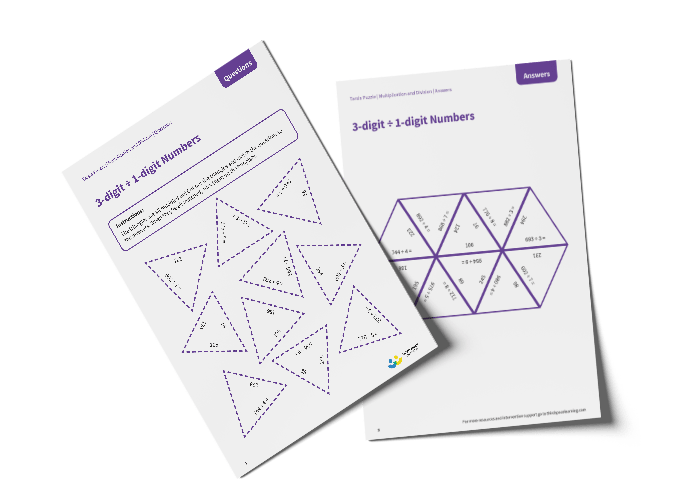# Tarsia Puzzle 3-digit Divide by 1-digit Numbers (Year 4)

The triangles in the tarsia puzzle have all been mixed up. In this 3-digit divided by 1-digit numbers tarsia puzzle, pupils will need to match the [topic] question to the correct answer to create one large hexagon.

This Year 4, 3-digit divide by 1-digit numbers tarsia puzzle worksheet contains:

• One question sheet
• One template sheet

Year 4

Division